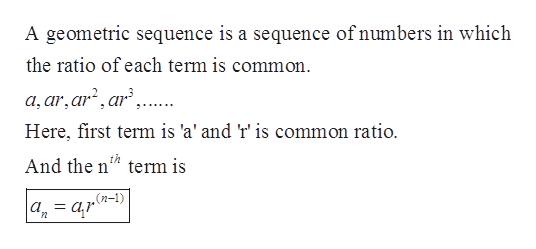# For Exercise, find the indicated term of a geometric sequence from the given information.a1 = 4 and a2 = 12. Find a6

Question
1 views

For Exercise, find the indicated term of a geometric sequence from the given information.

a1 = 4 and a2 = 12. Find a6

check_circle

Step 1

Given: the term of geometric sequence

Step 2

Concept used:help_outlineImage TranscriptioncloseA geometric sequence is a sequence of numbers in which the ratio of each term is common. a, ar,ar?, ar,.. Here, first term is 'a' and 'r' is common ratio. th And the n" term is (n-1) a, = a,r fullscreen
Step 3

First, we find the comm...

### Want to see the full answer?

See Solution

#### Want to see this answer and more?

Solutions are written by subject experts who are available 24/7. Questions are typically answered within 1 hour.*

See Solution
*Response times may vary by subject and question.
Tagged in

### Sequence and Series